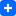# A solution for a Greedy Choice Algorithm Solution from TopCoder

``````
/**
* You are playing a computer game and a big fight is planned between two armies.
* You and your computer opponent will line up your respective units in two rows,
* with each of your units facing exactly one of your opponent's units and vice versa.
* Then, each pair of units, who face each other will fight and the stronger one will be
* victorious, while the weaker one will be captured. If two opposing units are equally strong,
* your unit will lose and be captured. You know how the computer will arrange its units,
* and must decide how to line up yours. You want to maximize the sum of the strengths of
* your units that are not captured during the battle.
* You will be given a int[] you and a int[] computer that specify the strengths of
* the units that you and the computer have, respectively. The return value should be an int,
* the maximum total strength of your units that are not captured.
*
* FROM TOP CODER
*
* your array
* {651, 321, 106, 503, 227, 290, 915, 549, 660, 115,
* 491, 378, 495, 789, 507, 381, 685, 530, 603, 394,
* 7, 704, 101, 620, 859, 490, 744, 495, 379, 781,
* 550, 356, 950, 628, 177, 373, 132, 740, 946, 609,
* 29, 329, 57, 636, 132, 843, 860, 594, 718, 849}
*
* computer array
* {16, 127, 704, 614, 218, 67, 169, 621, 340, 319,
* 366, 658, 798, 803, 524, 608, 794, 896, 145, 627,
* 401, 253, 137, 851, 67, 426, 571, 302, 546, 225,
* 311, 111, 804, 135, 284, 784, 890, 786, 740, 612,
* 360, 852, 228, 859, 229, 249, 540, 979, 55, 82}
*
* Returns: 25084
*
*/

import java.util.Arrays;
public class Main {

public void findMaxStrength(int[] computer, int[] player){
Arrays.sort(computer);
Arrays.sort(player);
int index = computer.length-1;
int smallIndex = 0;
for(int i = computer.length-1; i>=0; i--){
if(player[index] <= computer[i]){
shift(player, smallIndex, index);
index--;
}else{
index--;
}
}
}

public int[] shift(int[] a, int index1, int index2){
int e = a[index1];
for(int i = index1; i``````
``
`algorithmgreedyJavatop_coder`

# `Dynamic Programming Example - Find n.th Fibonacci number -`

`Dynamic programming is a concept that provides faster solutions for divide and conquer problems that have some number of overlapping sub problems. Generally this concept is used for optimization problems like finding longest common sub-sequence of two arrays.`

`However I used the concept in finding n th Fibonacci number. Here is the code that I used.`

``````public class Main {
int q;
public static void main(String args[]){
new Main().fibonacci(40);
}

public void fibonacci(int n){
ArrayList r = new ArrayList();

for(int i = 0; i < n+1; i++){
r.add(-1);
}
fibonacci(n, r);
System.out.println(q);
}

public int fibonacci(int n, ArrayList r){
if (r.get(n)>= 0){
return r.get(n);
}else if(n == 0){
q = 0;
}else if(n == 1){
q = 1;
}else{
q = fibonacci(n-1, r)+fibonacci(n-2, r);
}
r.set(n, q);
return q;
}
}
``````
``
`dynamic-programmingfibonacciJava`

# `Java Ant Compiling Problem Solution`

`I was working on a Strunt2 framework project and I needed to use ant in eclipse. I got a problem like this:`

``````BUILD FAILED: D:workspacespschemabuild.xml:37:
Unable to find a javac compiler;
com.sun.tools.javac.Main is not on the classpath.
Perhaps JAVA_HOME does not point to the JDK``````

`The solution is to go Window>>Preferences>>Ant>>Runtime than add the "tools.jar" file (somewhere in the JDK's folder) to jars that are in the Heading "Ant Home Entries".`

`That's it 🙂 have fun!`

``
`antcompileJavaproblem`